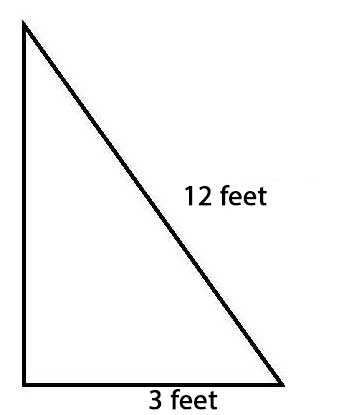# Applications of the Pythagorean Theorem, and the Midpoint Formula

Objective:
At the end of this lesson you will be able to:

1. Use right triangles to solve some simple applications.
2. Use the Midpoint Formula to find the point that is midway between two points on a line.

Prior Knowledge
Before you begin this lesson, you should know how to:

• Simplify and approximate square roots.
• Graph points and lines on the coordinate plane.
• Work with exponents.
• Understand the Pythagorean Theorem

### LESSON SUMMARY

Many practical problems can be solved using the Pythagorean Theorem. Here’s an example: Your suitcase is 22″ long, 14″ wide and 9″ deep. What is the length of the longest object you can lay flat on the bottom of your suitcase?

Using a diagram is often helpful in solving problems like this. The bottom of a suitcase is a rectangle. The longest object fits on the diagonal. We can represent this diagonal object with a dotted line. The sides of the suitcase and this dotted line form the sides of a right triangle.Use the Pythagorean Theorem to find the length of the diagonal. Substitute 22 for “a” and 14 for
b“. Solve the equation for “c“.

222 + 142 = c2
484 + 196 = c2
680 = c2
26.07 = c

The longest object you can fit on the bottom of your suitcase is a little more than 26 inches.

In the final part of this lesson, we work with the Midpoint Formula.

Using the Midpoint Formula, we can find the point midway between two coordinates. The
formula is:

$$\left(x,y\right)=\left(\frac{\left(x_1+x_2\right)}2,\frac{\left(y_1+y_2\right)}2\right)$$

To use the formula to find the point midway between (5, 10) and (-3, -5), we’ll label the points as
shown below.

$$\left(5,10\right)\;\;\;\;\left(-3,-5\right)$$
$$\left(x_1,\;y_1\right)\;\;\;\;\left(x_2,\;y_2\right)$$

Now substitute the coordinates in the formula and simplify the resulting equation.

$$\left(x,y\right)=\left(\frac{\left(5+\left(-3\right)\right)}2\right),\left(\frac{\left(10+\left(-5\right)\right)}2\right)$$
$$=\left(\frac22,\frac52\right)$$
$$=\left(1,2.5\right)$$

The point midway between (5, 10) and (-3, -5) is (1, 2.5).

Examples

These examples can help you master the new ideas in this lesson.

1. A ladder is leaning against a building. When the base of the ladder is 7.2 feet from the base of the building, it reaches to a point 9.6 feet high. If the ladder is moved so that its base is 3 feet from the wall, how far up the building will it reach?

Solution: First, find the length of the ladder. The ladder, the side of the building and the base (or ground) form a right triangle. In the diagram below, the hypotenuse is the ladder. The bottom of the ladder is 7.2 feet from the building. In this position, the top of the ladder reaches to 9.6 feet on the building.These distances are the legs in a right triangle, so we can substitute them into the Pythagorean theorem.

a2 + b2 = c2

9.62 + 7.22 = c2

92.16 + 51.84 = c2

144 = c2

12 = c

The ladder is 12 feet. Next, find the new height of the ladder when its base is moved 3 feet from the building. If it helps, use the diagram.The hypotenuse is 12 feet and one leg is 3 feet. Substitute these values into the Pythagorean Theorem. Then solve the resulting equation to find the length of the other leg, b.

a2 + b2 = c2

32 + b2 = 122

9 + b2 = 144

b2 = 135

b = 11.6

The top of the ladder is 11.6 feet high.

2. Use the points (-6, 5) and (8, -1) to find the point midway between the points.

Solution: Use the point (-6, 5) as (x1, y1) . Use (8, -1) as (x2, y2). Then substitute the points into the midpoint formula.

$$\left(x,y\right)=\left(\frac{\left(-6+8\right)}2\right),\left(\frac{\left(5+\left(-1\right)\right)}2\right)$$
$$=\left(\frac22,\frac42\right)$$
$$=\left(1,2\right)$$

The midpoint is (1, 2).

Practice Problems

These problems will give you more experience with the material in this lesson.

1. A decorator is planning to place ribbons diagonally on a rectangular tablecloth. If the
cloth measures 50″ by 70″, how long should each ribbon be cut? (Round your answer to
the nearest tenth.)
2. The trunk of Sam’s car is about 40″ wide and 45″ long. What is the longest object Sam
can fit into the bottom of the trunk? (Round off your answer to the nearest tenth.)
3. Find the point that is midway between the following points.
a) (-8, 3) and (-17, -9)
b) (2, 3) and (-5, 11)

1. 86”
2. 60.2”
3. (-12.5, 3)
4. (-1.5, 7)

R

R

R

R

R

R

R

R

R

R

R

R